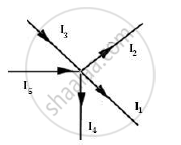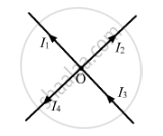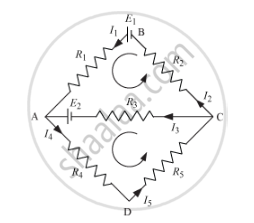# State the Two Kirchhoff’S Rules Used in Electric Networks. How Are There Rules Justified? - Physics

State the two Kirchhoff’s rules used in electric networks. How are there rules justified?

State Kirchhoff's rules. Explain briefly how these rules are justified.

#### Solution 1

Kirchhoff’s first rule:

In any electrical network, the algebraic sum of currents meeting at a junction is always zero.

∑I=0

In the junction below, let I1, I2, I3, I4 and I5 be the current in the conductors with directions as shown in the figure below. I5 and I3 are the currents which enter and currents I1, I2 and I4 leave.According to the Kirchhoff’s law, we have

(–I1) + (−I2) + I3 + (−I4) + I5 = 0 Or I1 + I2 + I4 = I3 + I5

Thus, at any junction of several circuit elements, the sum of currents entering the junction must equal the sum of currents leaving it. This is a consequence of charge conservation and the assumption that currents are steady, i.e. no charge piles up at the junction.

Kirchhoff’s second rule: The algebraic sum of changes in potential around any closed loop involving resistors and cells in the loop is zero. or

The algebraic sum of the e.m.f. in any loop of a circuit is equal to the algebraic sum of the products of currents and resistances in it.

Mathematically, the loop rule may be expressed as ∑E = ΣIR.

#### Solution 2

Kirchhoff’s First Law − Junction Rule

In an electrical circuit, the algebraic sum of the currents meeting at a junction is always zero.I1, I2 I3, and I4 are the currents flowing through the respective wires.

Convention:

The current flowing towards the junction is taken as positive.

The current flowing away from the junction is taken as negative.

I3 + (− I1) + (− I2) + (− I4) = 0

This law is based on the law of conservation of charge.

Kirchhoff’s Second Law − Loop Rule

In a closed loop, the algebraic sum of the emfs is equal to the algebraic sum of the products of the resistances and the currents flowing through them.For the closed loop BACB:

E1E2 = I1R1 + I2R2I3R3

E2 = I3R3 + I4R4 + I5R5

This law is based on the law of conservation of energy

Concept: Kirchhoff’s Rules
Is there an error in this question or solution?